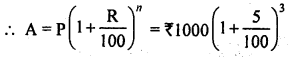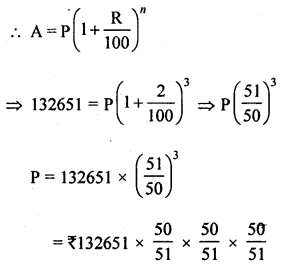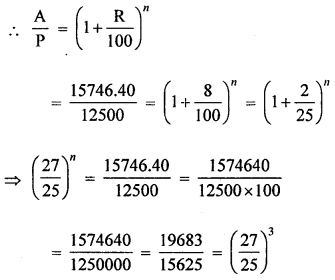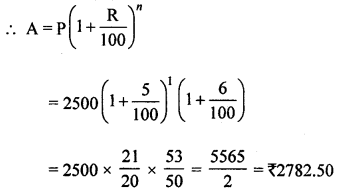## ML Aggarwal Class 8 Solutions for ICSE Maths Chapter 8 Simple and Compound Interest Check Your Progress

Question 1.
Find the amount and the compound interest on ₹5000 for 2 years at 6% per annum interest payable yearly.
Solution:
Principal (P) = ₹5000
Rate of interest (r) = 6% p.a.
Period (n) = 2 years∴ C.I. =A – P
= ₹5618 – ₹5000 = ₹618

Question 2.
Find the amount and the compounded interest on ₹7400 for 1 year at 5% per annum, interest payable half yearly.
Solution:
Principal (P) = ₹7400
Rate (R) = 5% p.a. or $$\frac{5}{2}$$ % half yearly
Period (n) = 1 year or 2 half yearsand C.I. = A – P = ₹7774.625 – ₹7400 = ₹374.625

Question 3.
Find the difference between C.I. and S.l. on sum of ₹5000 for 2 years at 8% per annum payable yearly.
Solution:
Principal = ₹5000
Rate (R) = 8% p.a.
Period (n) = 2 years∴ C.I. = A – P = ₹5832 – ₹5000 = ₹832
Now difference between C.I. and S.l.
= ₹832 – ₹800 = ₹32

Question 4.
Find the amount and compound interest on ₹10000 for $$1 \frac{1}{2}$$ years at 10% per annum, compounded half yearly. Would this interest be more than the interest he would get if it was compounded annually?
Solution:
Principal (P) = ₹10000
Rate (R) = 10% p.a. or 5% half yearly
Period (n) = $$1 \frac{1}{2}$$ years or 3 half years∴ and C.I. = ₹11550 – 10000 = ₹1550
Yes, the interest compounded half yearly is more than yearly.

Question 5.
What sum invested for $$1 \frac{1}{2}$$ years compounded half yearly at the rate of 4% p.a. will amount to ₹ 132651 ?
Solution:
Amount (A) = ₹ 132651
Rate (R) = 4% p.a. or 2% half yearly
Period (n) = $$1 \frac{1}{2}$$ years or 3 half years= ₹125000

Question 6.
Find the time (in years) in which ₹ 12500 will produce ₹3246.40 as compound interest at 8% per annum, compounded annually.
Solution:
Principal (P) = ₹12500
C.I. = ₹3246.40
∴ Amount = P + C.I. = 12500 + 3246.40
= ₹ 5746.40
Rate (R) = 8% p.a.Comparing, we get n = 3
∴ Time = 3 years

Question 7.
Find the amount and compound interest on ₹2500 in 2 years if the rate are 5% and 6% for the successive years.
Solution:
Principal (P) = ₹2500
Rate (R) = 5% and 6% for 2 successive yearsand C.I. =A – P = ₹2782.50 – 2500 = ₹282.50